# a) suppose that the nxn matrix A has its n eigenvalues arranged in decreasing order of absolute size, so that  >>....

a) suppose that the nxn matrix A has its n eigenvalues arranged in decreasing order of absolute size, so that>>.... each eigenvalue has its corresponding eigenvector, x1,x2,...,xn. suppose we make some initial guess y(0) for an eigenvector. suppose, too, that y(0) can be written in terms of the actual eigenvectors in the form y(0)=alpha1.x1 +alpha2.x2 +...+alpha(n).x(n), where alpha1, alpha2, alpha(n) are constants. by considering the "power method" type iteration y(k+1)=Ay(k) argue that (see attached image)

b) from an nxn matrix A, with eigenvalues and eigenvectors in part a, show that inverse matrix A^-1 has the same eigenvectors as A, but that its eigenvalues are 1/lamda1, 1/lamda2,...,1/lamda(n). what will the power method do, if applied to this inverse matrix A^-1

c)the nxn matrix B is defined to be similar to nxn matrix A if B=P^(-1)AP. here P is a non-singular nxn matrix. show that B has the same eigenvalues as A.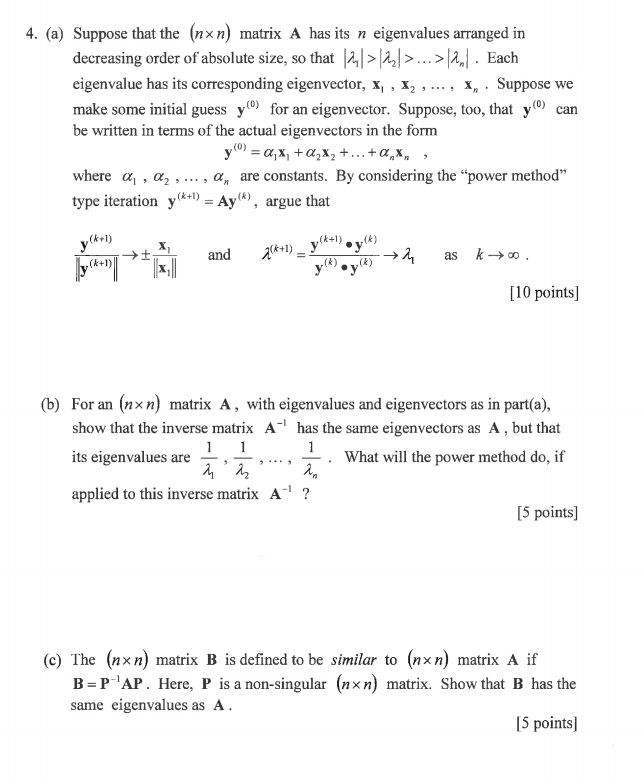12
An
4. (a) Suppose that the (nxn) matrix A has its n decreasing order of absolute size, so that |2,| > |2,| >... >|2|. Each eigenvalue has its corresponding eigenvector, x, , x2 , ..., x, Suppose we make some initial guess y0) for an eigenvector. Suppose, too, that y(0) can be written in terms of the actual eigenvectors in the form eigenvalues arranged in у 3 а, х, +а,х, +...+a,х, where a a2, ... , a, are constants. By considering the "power method" type iteration yA+1) = Ay(*), argue that (k) уt-) (k+1) (k+1) and as yy) (k) (k) (k+1) [10 points] (b) For an (nxn) matrix A, with eigenvalues and eigenvectors as in part(a), show that the inverse matrix A1 has the same eigenvectors as A , but that 1 its eigenvalues are What will the power method do, if applied to this inverse matrix A1 ? [5 points] (c) The (nxn) matrix B is defined to be similar to B PAP. Here, P is a non-singular (nxn) matrix. Show that B has the same eigenvalues as A (nxn) matrix A if [5 points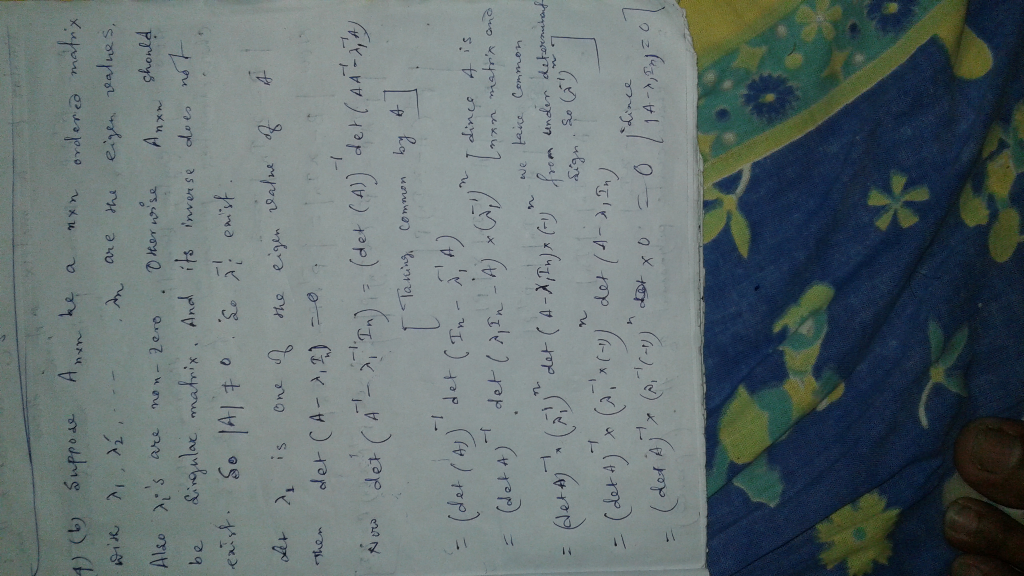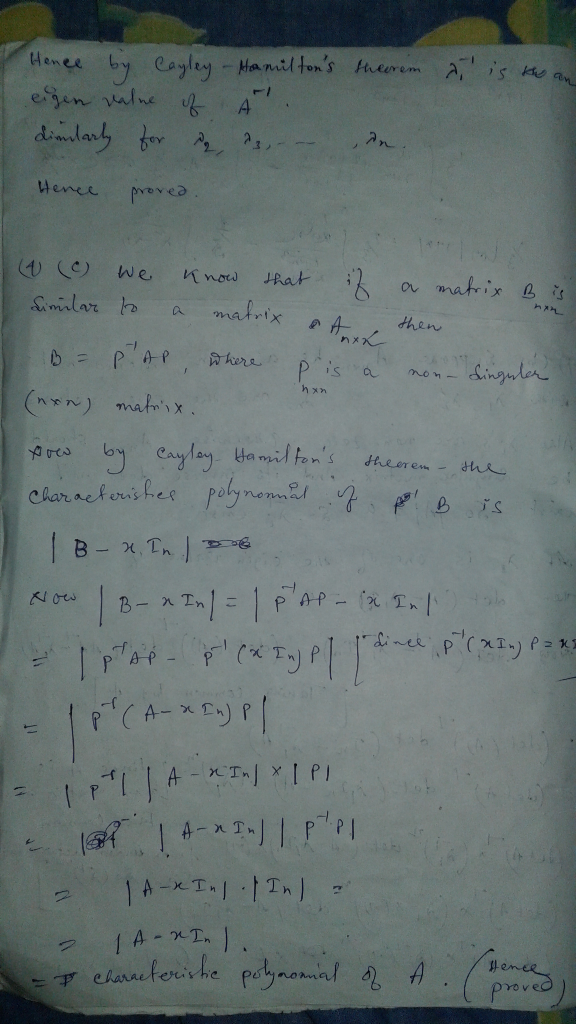##### Add Answer of: a) suppose that the nxn matrix A has its n eigenvalues arranged in decreasing order of absolute size, so that  >>....
Similar Homework Help Questions
• ### (1 point) Suppose that the matrix A has the following eigenvalues and eigenvectors: A1 = 4...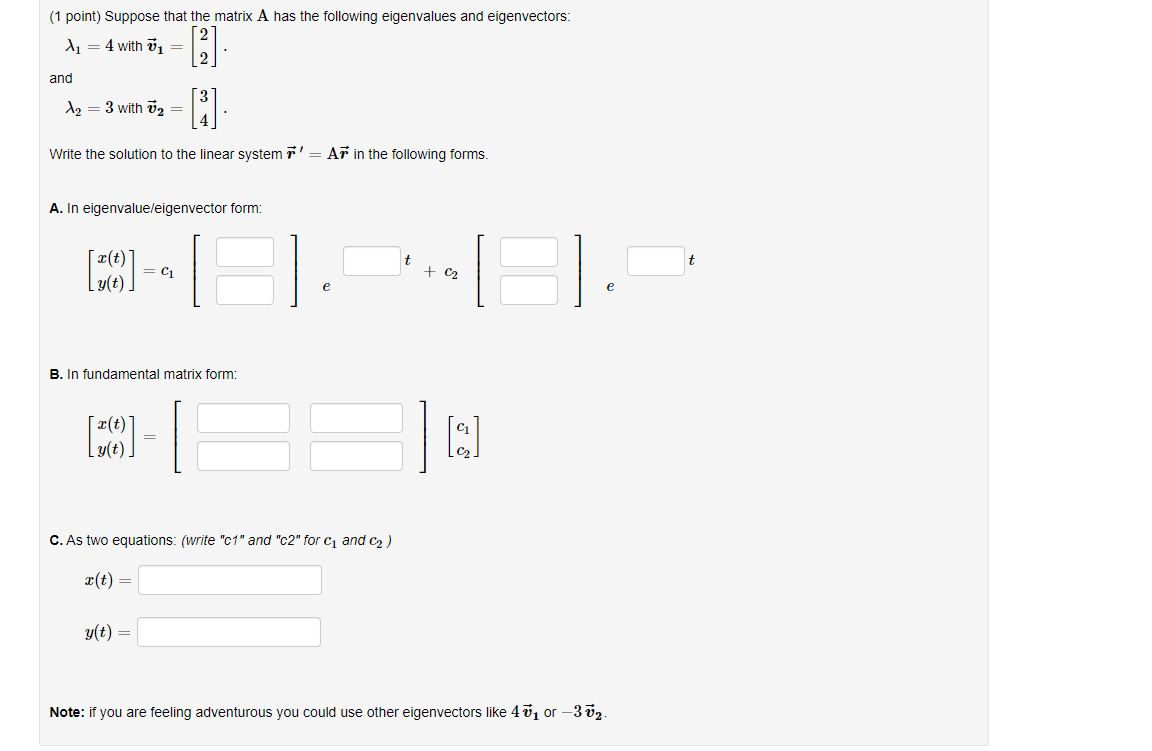(1 point) Suppose that the matrix A has the following eigenvalues and eigenvectors: A1 = 4 with = and  [i] Az = 3 with Ū2 = Write the solution to the linear system r' = Ar in the following forms. A. In eigenvalue/eigenvector form: t (10) -- + C2 e e B. In fundamental matrix form: (39) - g(t). C. As two equations: (write "c1" and "c2" for C and C2) X(t) = g(t) = Note: if you are...

• ### Question 8 (20 points). (a) Suppose that M is an nxn matrix such that M =...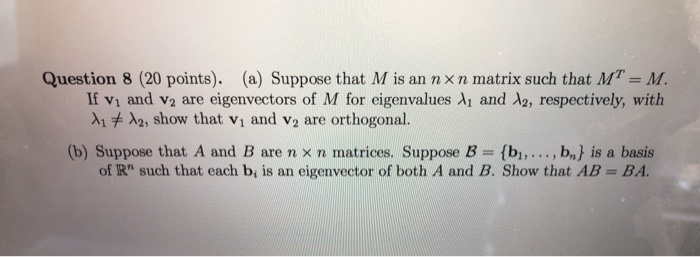Question 8 (20 points). (a) Suppose that M is an nxn matrix such that M = M. If V, and V2 are eigenvectors of M for eigenvalues X, and 12, respectively, with X1 + X2, show that v, and V, are orthogonal. (b) Suppose that A and B are n x n matrices. Suppose B = {bı,..., b} is a basis of R" such that each b, is an eigenvector of both A and B. Show that AB = BA.

• ### Suppose that the matrix A A has the following eigenvalues and eigenvectors: (1 point) Suppose that...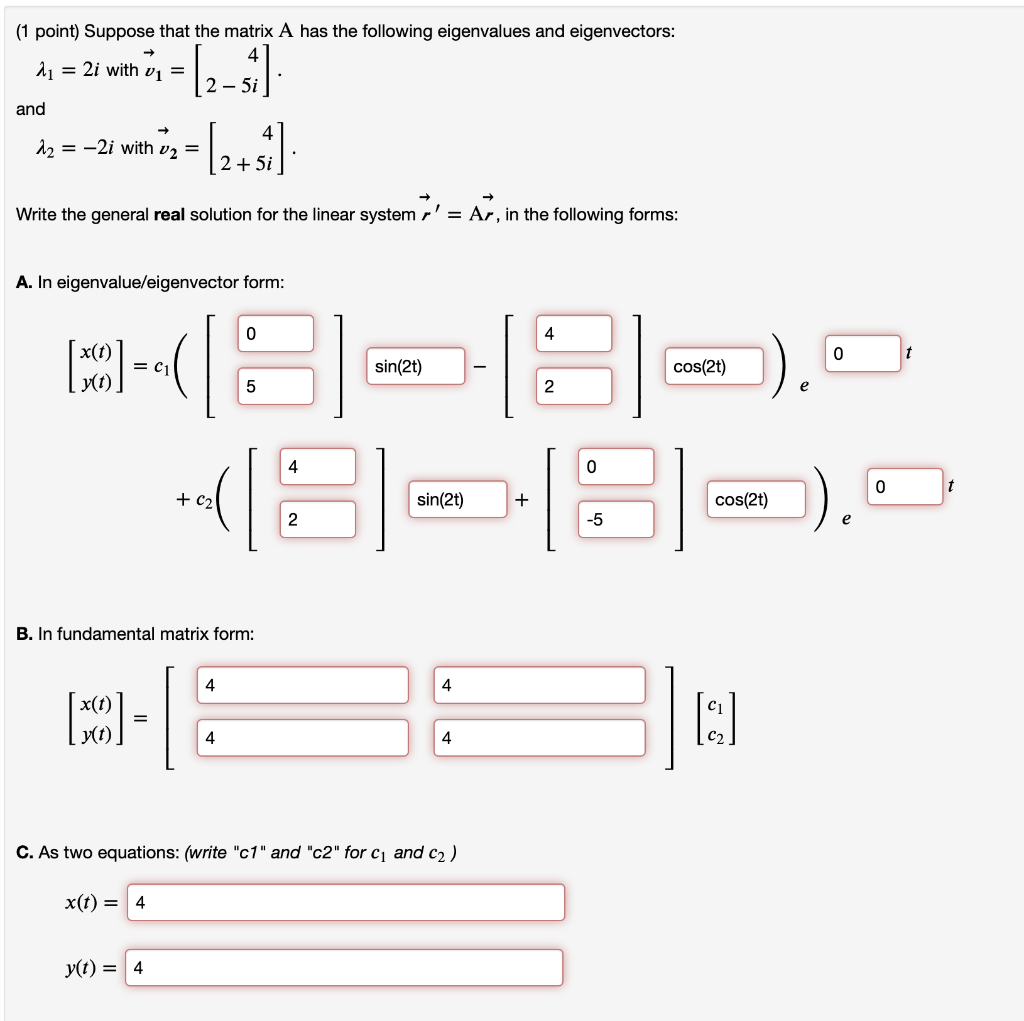Suppose that the matrix A A has the following eigenvalues and eigenvectors: (1 point) Suppose that the matrix A has the following eigenvalues and eigenvectors: 2 = 2i with v1 = 2 - 5i and - 12 = -2i with v2 = (2+1) 2 + 5i Write the general real solution for the linear system r' = Ar, in the following forms: A. In eigenvalue/eigenvector form: 0 4 0 t MODE = C1 sin(2t) cos(2) 5 2 4 0 0...

• ### Let А and B be similar nxn matrices. That is, we can write A = CBC-...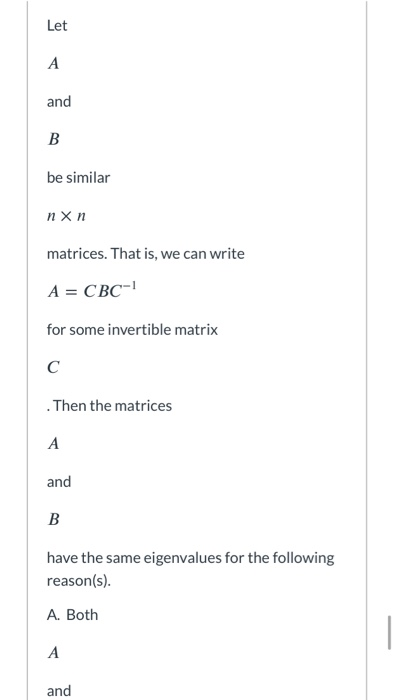Let А and B be similar nxn matrices. That is, we can write A = CBC- for some invertible matrix с Then the matrices A and B have the same eigenvalues for the following reason(s). A. Both А and A. Both А and B have the same characteristic polynomial. B. Since A = CBC-1 , this implies A = CC-B = IB = B and the matrices are equal. C. Suppose that 2 is an eigenvalue for the matrix B...

• ### (1 point) Suppose that the matrix A has the following eigenvalues and eigenvectors: 4 = 2...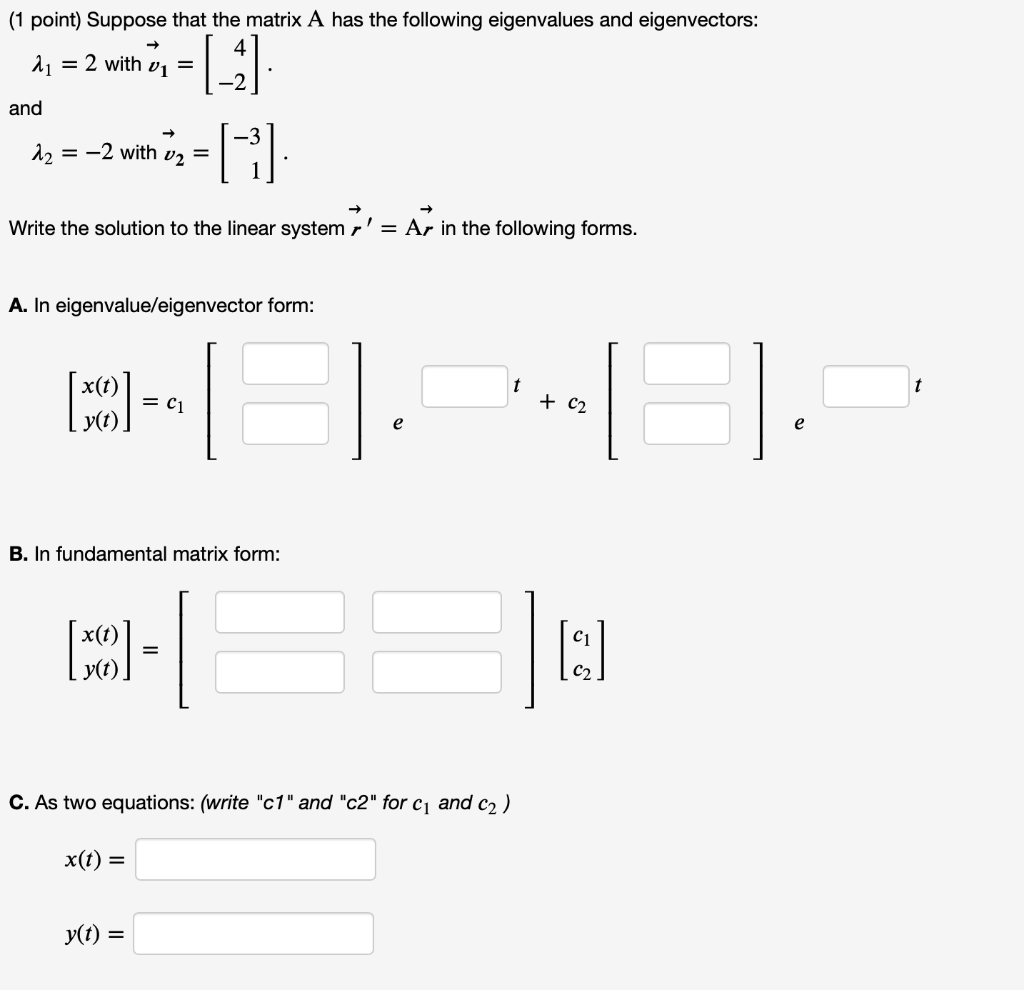(1 point) Suppose that the matrix A has the following eigenvalues and eigenvectors: 4 = 2 with vi = and |_ G 12 = -2 with v2 = Write the solution to the linear system r' = Ar in the following forms. A. In eigenvalue/eigenvector form: x(t) (50) = C1 + C2 e e B. In fundamental matrix form: (MCO) = I: C. As two equations: (write "c1" and "c2" for C1 and c2) x(t) = yt) =

• ### 5. The following matrix B has known eigenvalues λ1-1 and λ2-6. 10a-1 B-0b-23 c30 0 Where...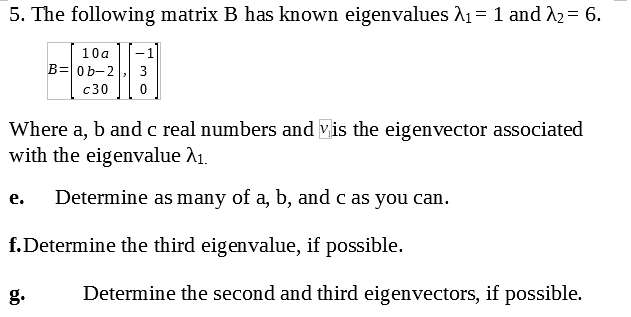5. The following matrix B has known eigenvalues λ1-1 and λ2-6. 10a-1 B-0b-23 c30 0 Where a, b and c real numbers and vis the eigenvector associated with the eigenvalue A1. e. Determine as many of a, b, and c as you can. f.Determine the third eigenvalue, if possible. g.Determine the second and third eigenvectors, if possible.

• ### 0 -2 - The matrix A -11 2 2 -1 has eigenvalues 5 X = 3,...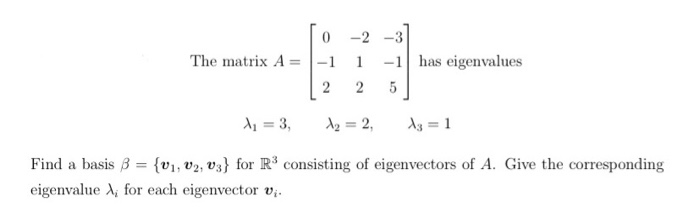0 -2 - The matrix A -11 2 2 -1 has eigenvalues 5 X = 3, A2 = 2, 13 = 1 Find a basis B = {V1, V2, v3} for R3 consisting of eigenvectors of A. Give the corresponding eigenvalue for each eigenvector vi.

• ### True or False? 1. If σ is a singular value of a matrix A, then σ is an eigenvalue of ATA Answer: 2. Every matrix has the same singular values as its transpose Answer: 3. A matrix has a pseudo-inverse...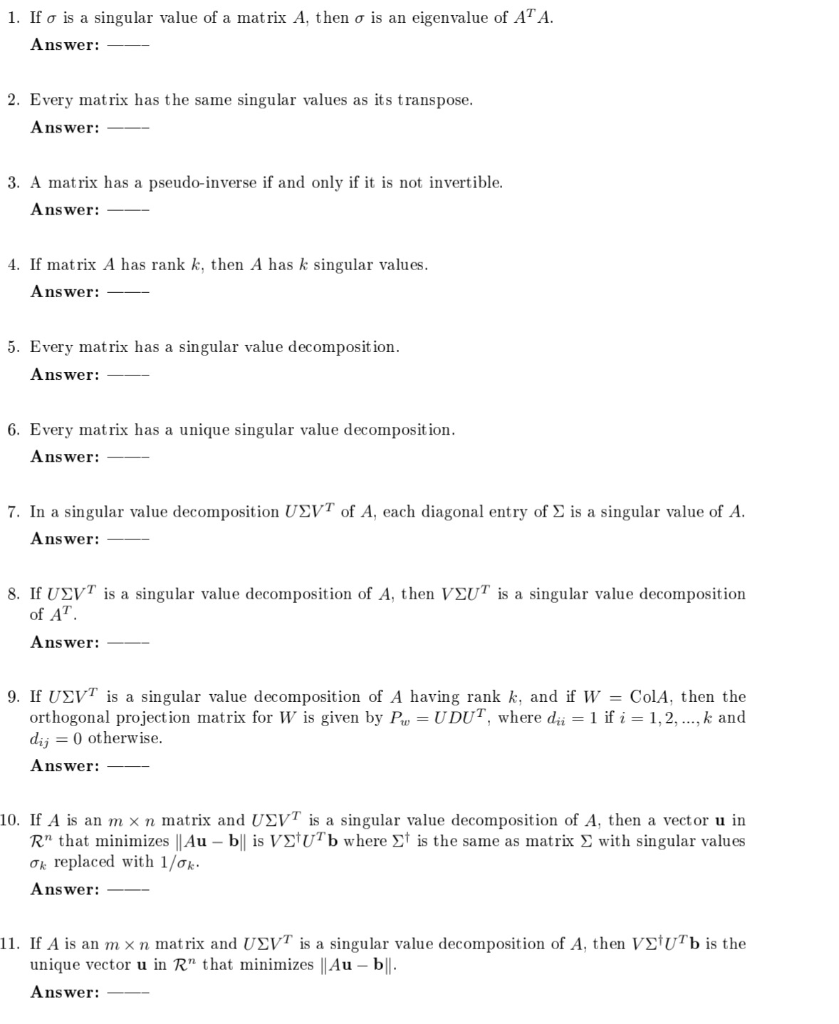True or False? 1. If σ is a singular value of a matrix A, then σ is an eigenvalue of ATA Answer: 2. Every matrix has the same singular values as its transpose Answer: 3. A matrix has a pseudo-inverse if and only if it is not invertible. Answer: 4. If matrix A has rank k, then A has k singular values Answer:_ 5. Every matrix has a singular value decomposit ion Answer:_ 6. Every matrix has a unique singular...

• ### (1 point) Suppose that the matrix A has repeated eigenvalue with the following eigenvector and generalized...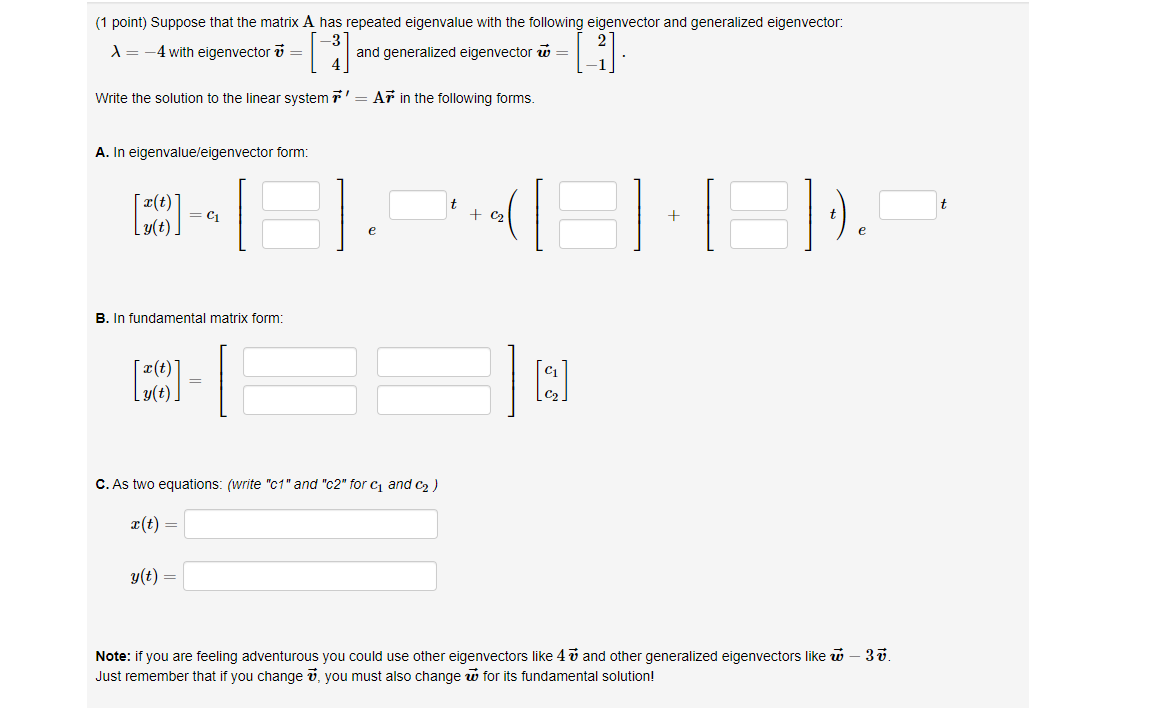(1 point) Suppose that the matrix A has repeated eigenvalue with the following eigenvector and generalized eigenvector: X= -4 with eigenvector v = and generalized eigenvector ū= [] (-1) Write the solution to the linear system r' = Ar in the following forms. A. In eigenvalue/eigenvector form: t t [CO] = C1 + C2 + I g(t). e . - 1 B. In fundamental matrix form: [CO] C. As two equations: (write "c1" and "c2" for 1 and 2) X(t)...

• ### code in matlab 1. [2+1+1pt] Power Method and Inverse Iteration. (a) Implement the Power Method. Use...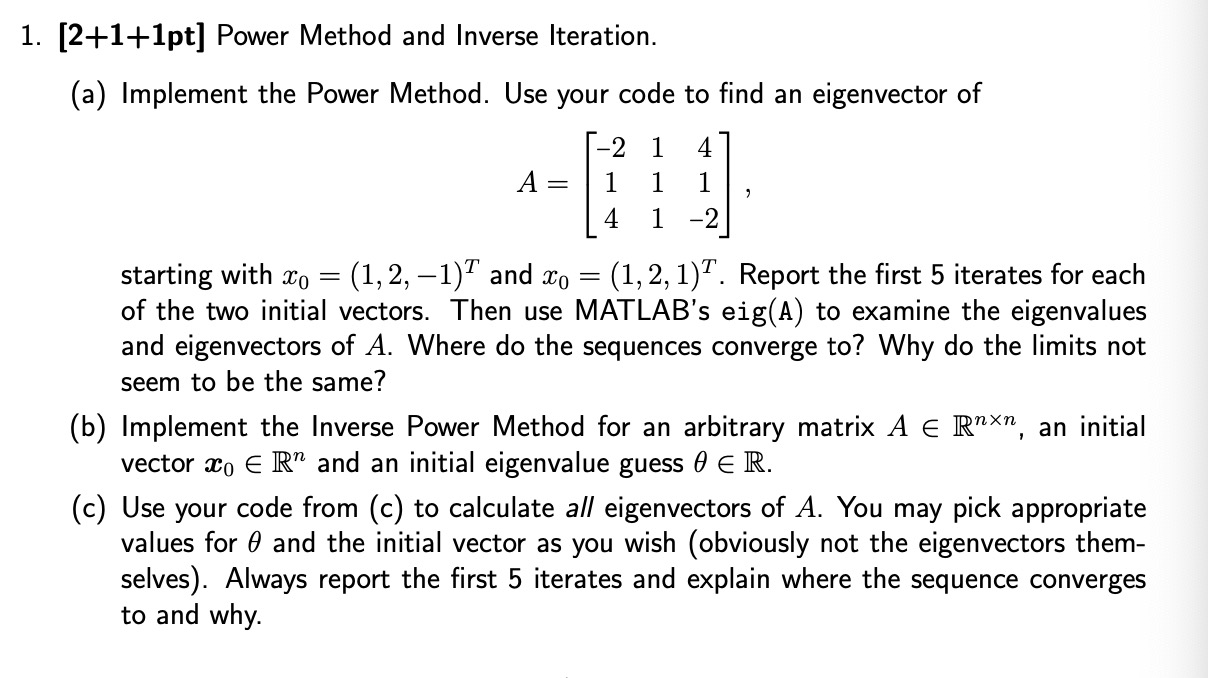code in matlab 1. [2+1+1pt] Power Method and Inverse Iteration. (a) Implement the Power Method. Use your code to find an eigenvector of -2 1 4 A= 1 1 2 4 1 -2 starting with Xo = (1, 2, -1)7 and Xo = (1, 2, 1)7. Report the first 5 iterates for each of the two initial vectors. Then use MATLAB's eig(A) to examine the eigenvalues and eigenvectors of A. Where do the sequences converge to? Why do the limits...

Free Homework App